<% tit="Feedback Form" cmd=request("cmd") if cmd>"" then errmsg="Invalid email. Please correct your email" ton=request("ton") frn=request("frn") fre=request("fre") sbj=request("sbj") sba=request("sba") Priority=request("Priority") msg=request("msg") x=instr(fre,"@") x2=0 if x>2 then x2=instr(x+1,fre,".",1) if x2>x then if not mxlookup(mid(fre,x+1)) then x2=0 end if if x2=0 then cmd="" else c=vbcrlf toe=ton &"@zenith.com.sg" sb2=sbj if sba>"" then sb2=sba &": "& sb2 sndmail fre,frn, toe,ton, fre, sb2, "To "& ton &","& c & c & msg & c & c &"From "& frn &","& c & fre & c&c &"Send from URL: "& request("rfr") errmsg="" tit="Feedback Confirmation" end if end if FUNCTION MXLookup(host) Dim o,s Set o=Server.CreateObject("Microsoft.XMLHTTP") o.Open "Get","http://examples.softwaremodules.com/IntraDns.asp?domainname=" & host & "&Submit=Submit&t_mx=1", False o.Send s = o.ResponseText s = Mid(s,InStr(s,"(MX) for "),100) s = Mid(s,Instr(s,". Items Returned: ")+35,1) MxLookup= CInt(s)>0 END FUNCTION %> <%=tit%>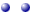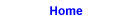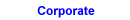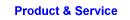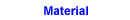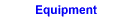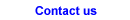## Enquiry / Feedback Form

<% if request("cmd")="" then rfr=request("rfr") if rfr="" then rfr=Request.ServerVariables("HTTP_REFERER")%>You may like to send us your query, view or suggestions, to enhance your surfing experience.

Thank you for taking the time to feedback to us.

 To <% a1="" a2="" a3="" if Request("To")="Sales" then a1=" selected" elseif Request("To")="Salesvn" then a2=" selected" else a3=" selected" end if%> Accounts Department Admin Department >Sales Department >Sales (Vietnam Branch) >WebMaster Name "> eMail "> Example of a typical email address would be myID@singnet.com.sg Subject "> Subject Area Enquiry Comments/Suggestion Products Services Web Link Issue Web Content Issue Web Design Issue >Web Tips General Priority Highest High Normal Low Lowest Comment / Enquiry

<% if errmsg>"" then response.write "
"& errmsg &"
" else%>The following information has been submitted:
 To <%=toe%> From <%if frn="" then response.write fre else response.write frn&" ("&fre&")" end if%> Subject <%=sbj%> Subject Area <%=sba%> Message <%=Replace(msg, vbcrlf, "")%>

Thank you for taking the time to complete the email form.

If a response is expected from this email, we will response to you the soonest possible.

<% end if %>